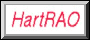# Analemmatic Sundial Mathematics

```
/* analemmatic sundial computations for use in a program or speadsheet
with signs appropriate for southern hemisphere

longcor = longitude correction
e.g. longcor for Gauteng at 28 deg east = -8 minutes = -2 degrees,
Southern African Standard Time being for 30 degrees east time zone

lat = latitude,
e.g. Gauteng latitude = 26 deg south = -26 degrees

data needed for the analemma (dateline):
Sundec = declination of the Sun in degrees at date
ETmin  = equation of time at date, in minutes
*/

date      Sundec       ETmin
Jan01     -23.067       -3.70
Jan15     -21.242       -9.27
Feb01     -17.333      -13.70
Feb15     -12.904      -14.13
Mar01      -7.817      -12.40
Mar15      -2.401       -9.03
Apr01       4.300       -4.00
Apr15       9.526       -0.13
May01      14.900        3.00
May15      18.706        3.68
Jun01      21.967        2.30
Jun15      23.277       -0.37
Jul01      23.150       -3.60
Jul15      21.625       -5.92
Aug01      18.167       -6.10
Aug15      13.942       -4.55
Sep01       8.500        0.00
Sep15       3.284        4.65
Oct01      -2.950       10.30
Oct15      -8.266       14.13
Nov01     -14.233       16.40
Nov15     -18.309       15.47
Dec01     -21.716       10.90
Dec15     -23.228        5.05

/* two constants are needed:
scale = scale size, 2.5 is good size in metres for walk on sundial
but 1.5 - 2.0 will be better for young people*/
scale=2.5;

/* dtr = degrees to radians conversion for libraries wanting trig functions
in radians; set to 1.0 if trig functions use degrees */
dtr=0.017453293;

/* time line, where Tdeg = standard time in degrees (1 degree = 4 minutes),
let Tdeg run from 06h00 to 18h00 at half hour intervals */

/* Xtime = M sin(T +/- longitude correction in degrees) */
Xtime=-scale*sin((Tdeg+longcor)*dtr);

/* Ytime = M sin(lat) cos(T +/- long. corr.) */
Ytime=-scale*sin(-lat*dtr)*cos((Tdeg+longcor)*dtr);

/* analemma Y axis = M tan(Sundec) cos(lat) */
Yan=-scale*tan(Sundec*dtr)*cos(lat*dtr);

/* analemma X axis = -M ETmin converted to degrees */
Xan=+scale*(ETmin/4.0*dtr);

```

This is a southern hemisphere example, for Gauteng Province in South Africa. Note the signs for Xtime, Ytime, Xan, Yan.

```/* sundlgtg.hst - Gauteng
longitude correction for 28 deg east = -8 mins = -2 deg
latitude = 26 deg south
X = M sin(T +/- Longitude correction in degrees)
Y = M sin(lat) cos(T +/- long. corr.)
where T = SAST in degrees */
Xtime=-2.5*sin((Tdeg-2)*0.017453293);
Ytime=-2.5*sin(-26*0.017453293)*cos((Tdeg-2)*0.017453293);
/* analemma Y axis = M tan(dec) cos(lat) */
Yan=-2.5*tan(Dec*0.017453293)*cos(-26*0.017453293);
/* analemma X axis = -M ETmin -> degrees */
Xan=+2.5*(ETmin/4.0*0.017453293);
```

This is a northern hemisphere example, for Brocton in England. Note the signs for Xtime, Ytime, Xan, Yan.

```/* sundlBRO.hst - for Brocton, England
longitude correction at 2d 02' 20" west = 8.156 mins = 2.039 deg
latitude = 52d 47' 40" north  = 52.778 deg
X = M sin(T +/- Longitude correction in degrees)
Y = M sin(lat) cos(T +/- long. corr.)
where T = Time Zone standard time in degrees */
Xtime=+2.5*sin((Tdeg-2.039)*0.017453293);
Ytime=+2.5*sin(+52.778*0.017453293)*cos((Tdeg-2.039)*0.017453293);
/* analemma Y axis = M tan(dec) cos(lat) */
Yan=+2.5*tan(Dec*0.017453293)*cos(+52.778*0.017453293);
/* analemma X axis = -M ETmin -> degrees */
Xan=-2.5*(ETmin/4.0*0.017453293);
```

www.hartrao.ac.za/other/sundial/sundlmath.html
Last updated 2000/05/08 by M Gaylard e-mail: mike@hartrao.ac.za